# IC Engine - 1

## 20 Questions MCQ Test Mock Test Series for SSC JE Mechanical Engineering | IC Engine - 1

Description
Attempt IC Engine - 1 | 20 questions in 12 minutes | Mock test for SSC preparation | Free important questions MCQ to study Mock Test Series for SSC JE Mechanical Engineering for SSC Exam | Download free PDF with solutions
QUESTION: 1

### Which gas will produce the highest efficiency in an ideal Otto cycle for same compression ratio?

Solution:

The efficiency of Otto cycle is given as η=1−(1r)γ−1η=1−(1r)γ−1

Higher the value of γ more will be the efficiency for same compression ratio. The value of γ for the different type of gases is given below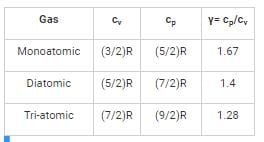γ monoatomic > γdiatomic > γtriatomic

So out of given options Helium is mono atomic, so efficiency will be highest for Helium for a given compression ratio.

QUESTION: 2

### Exhaust gas recirculation has the disadvantage of

Solution:

Although Exhaust Gas Recirculation (EGR) reduces the maximum temperature in the combustion chamber but it also lowers the overall combustion efficiency. Increase in EGR results in some cycle partial burns and in the extreme, total misfires. Thus, by using EGR to reduce NOX emissions, a costly price of increased HC emission and lower thermal efficiency must be paid.

QUESTION: 3

### Octane number of gasoline is a measure of its:

Solution:

Octane rating or octane number is a measure of the resistance of gasoline and other fuels to detonation (engine knocking) in spark-ignition internal combustion engines. Higher octane rating means higher knocking resistance tendency.

QUESTION: 4

The engine of a car has three cylinder with total displacement of 770 cc. The compression ratio is 8.7. What is the clearance volume of each cylinder?

Solution: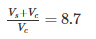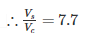Now total displaced volume for three cylinders is 770 cc. So for one cylinder the VS = 770/3 cc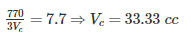QUESTION: 5

The magneto in an automobile is basically a/an

Solution:

magneto is a special type of electric generator. It is mounted on the engine and replaces all the components of the coil ignition system except the spark plug. A magneto, when rotated by the engine, is capable of producing very high voltage and does not need a battery as a source of external energy.

QUESTION: 6

Knocking in spark ignition engine can be reduced by

Solution:

Factors affecting knocking in SI engine:

i) Compression ratio:- Higher compression ratio will result in higher temperature of the charge, tendency to knock will increase.

ii) Inlet air temperature:- The effect of inlet air temp is same as compression ratio. High inlet temp of the air fuel mixture will increase knocking.

iii) Advancing the spark:- Advancing the spark will result in more compression during sparking. The increase in temperature during the sparking will be higher. Hence tendency to knock will increase and so by retarding the spark, the tendency to knock will decrease.

iv) Coolant water temperature:- By increasing coolant water temperature, less heat will be carried from the engine which will increase the engine temperature. So the knocking will increase.

QUESTION: 7

Which is the wrong assumption for calculation of air standard efficiency?

Solution:

To simplify analysis, the following assumptions are made for calculation of air standard efficiency:

1. The working fluid is air, which continuously circulates in a closed loop and always behaves as an ideal gas.

2. All the processes that make up the cycle are internally reversible.

3. The combustion process is replaced by a heat - addition process from an external source.

4. The exhaust process is replaced by a heat - rejection process that restores the working fluid to its initial state.

QUESTION: 8

Orsat apparatus is used to determine products of

Solution:

Orsat apparatus is used to analyse the combustion products. It measure the volume fractions of CO2 and O2 in a sample of dry combustion products from the continuous combustion unit.

QUESTION: 9

A four-stroke petrol engine at full load delivers 50 kW. It requires 8.5 kW to rotate it without load at the same speed. Find its mechanical efficiency (in %) at half load.

Solution:

Mechanical efficiency at half load =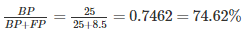QUESTION: 10

Detonation in the SI engine becomes severe during

Solution:

Detonation in SI engine will become severe at high load and low speed because at higher loads, the temperature of cylinder and combustion chamber walls will be higher and hence temperature of the end charge will be more which increases knocking tendency in SI engine.

And at lower engine speed, longer will be the absolute time for the flame to traverse the cylinder which increases the time available for pre flame reactions hence the tendency to knock increases.

QUESTION: 11

Brake specific fuel consumption is defined as

Solution:

The meaning of bsfc is how much fuel is consume in one hour to produce one kilowatt brake power(i.e. power available at engine shaft not power produce into engine block).

It is used to measure fuel efficiency and also to compare internal combustion engine on basis of shaft power.

QUESTION: 12

The four process of the Carnot cycles are

Solution:

Carnot cycle consists of two reversible isothermal and two isentropic process.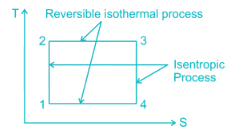QUESTION: 13

An ideal gas with heat capacity ratio of 2 is used in an ideal Otto-cycle which operates between minimum and maximum temperatures of 200 K and 1800 K. What is the compression ratio of the cycle for maximum work output?

Solution:

Heat capacity ratio, γ = Cp/Cv = 2

For maximum work output for Otto cycle: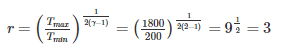QUESTION: 14

What is the number of cycles completed per second for a four stroke diesel engine running at 6000 rpm?

Solution:

Engine speed = 6000 RPM.

One cycle in four stroke requires two revolutions. So Number of cycles per minute = 6000/2 = 3000 cycles.

So number of cycles per minute = 3000/60 = 50 cycles

QUESTION: 15

The indicator on an engine is used to determine:

Solution:

The device which measures the variations of the pressure in the cylinder over a part or full cycle is called an indicator and the plot of such information obtained is called an indicator diagram. This shows the mean effective pressure and indicated horse power.

QUESTION: 16

Morse test is carried out to determine ______ of an engine:

Solution:

Morse test is a very useful test designed for multi cylinder engines. Its primary objective is to determine the indicated power developed by each cylinder. The indicated power is the sum of break horse power plus the frictional power losses.

QUESTION: 17

By higher octane number of SI fuel, it means that fuel has

Solution:

Higher octane is related only to longer ignition delay which prevents knocking. High octane fuel itself don’t increase the power or enhance any other property of fuel

QUESTION: 18

Pour point of fuel oil is the

Solution:

The pour point in that temperatures just about which the oil sample will not flow under certain prescribed conditions. In other words, pour point of a liquid is the temperature below which the liquid loses its flow characteristics.

QUESTION: 19

The combustion in a compression ignition engine is ________.

Solution:

Homogeneous compression ignition is a form of internal combustion in which air and fuel are well mixed at the point of combustion. On the other hand, in heterogenous combustion engine, the air and fuel are not mixed till the point of combustion. In CI engines or diesel engine, only air is compressed and then the fuel is injected at the last stage. So no proper mixing before combustion takes place. So the CI engines are heterogenous combustion engines.

QUESTION: 20

The process scavenging is relevant in

Solution:

Scavenging is the process whereby air at a pressure greater than that of atmospheric pressure is used to push the exhaust gas out of the cylinder of an engine. Unlike the 4 stroke engine, a two stroke diesel engine does not use the piston to push out the exhaust gas, instead, air enters the cylinder around bottom dead centre and sweeps or scavenges the exhaust gas from the cylinderUse Code STAYHOME200 and get INR 200 additional OFF Use Coupon Code